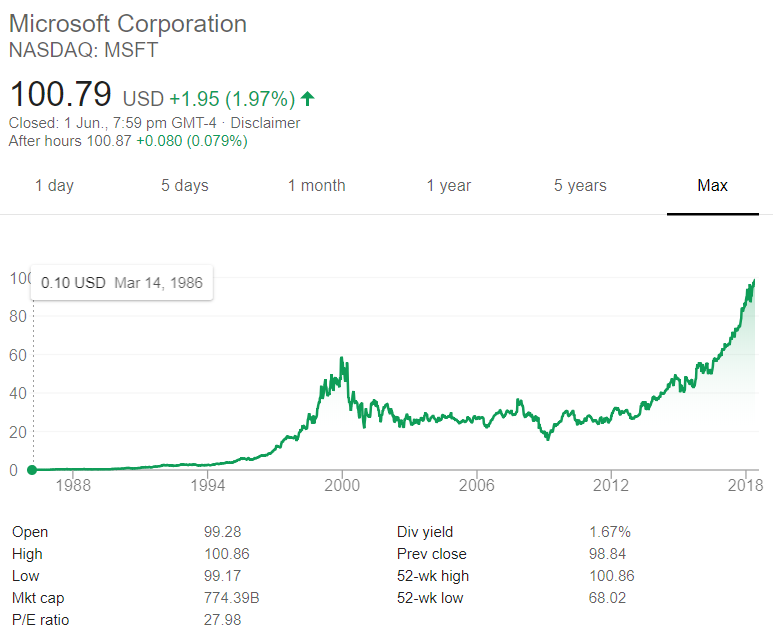# Fight Finance

#### CoursesTagsRandomAllRecentScores

Your friend just bought a house for $400,000. He financed it using a$320,000 mortgage loan and a deposit of $80,000. In the context of residential housing and mortgages, the 'equity' tied up in the value of a person's house is the value of the house less the value of the mortgage. So the initial equity your friend has in his house is$80,000. Let this amount be E, let the value of the mortgage be D and the value of the house be V. So $V=D+E$.

If house prices suddenly fall by 10%, what would be your friend's percentage change in equity (E)? Assume that the value of the mortgage is unchanged and that no income (rent) was received from the house during the short time over which house prices fell.

Remember:

$$r_{0\rightarrow1}=\frac{p_1-p_0+c_1}{p_0}$$

where $r_{0-1}$ is the return (percentage change) of an asset with price $p_0$ initially, $p_1$ one period later, and paying a cash flow of $c_1$ at time $t=1$.

Which one of the following bonds is trading at a discount?

A five year bond has a face value of $100, a yield of 12% and a fixed coupon rate of 6%, paid semi-annually. What is the bond's price? If the current AUD exchange rate is USD 0.9686 = AUD 1, what is the European terms quote of the AUD against the USD? Some financially minded people insist on a prenuptial agreement before committing to marry their partner. This agreement states how the couple's assets should be divided in case they divorce. Prenuptial agreements are designed to give the richer partner more of the couples' assets if they divorce, thus maximising the richer partner's: The boss of WorkingForTheManCorp has a wicked (and unethical) idea. He plans to pay his poor workers one week late so that he can get more interest on his cash in the bank. Every week he is supposed to pay his 1,000 employees$1,000 each. So $1 million is paid to employees every week. The boss was just about to pay his employees today, until he thought of this idea so he will actually pay them one week (7 days) later for the work they did last week and every week in the future, forever. Bank interest rates are 10% pa, given as a real effective annual rate. So $r_\text{eff annual, real} = 0.1$ and the real effective weekly rate is therefore $r_\text{eff weekly, real} = (1+0.1)^{1/52}-1 = 0.001834569$ All rates and cash flows are real, the inflation rate is 3% pa and there are 52 weeks per year. The boss will always pay wages one week late. The business will operate forever with constant real wages and the same number of employees. What is the net present value (NPV) of the boss's decision to pay later? Many Australian home loans that are interest-only actually require payments to be made on a fully amortising basis after a number of years. You decide to borrow$600,000 from the bank at an interest rate of 4.25% pa for 25 years. The payments will be interest-only for the first 10 years (t=0 to 10 years), then they will have to be paid on a fully amortising basis for the last 15 years (t=10 to 25 years).

Assuming that interest rates will remain constant, what will be your monthly payments over the first 10 years from now, and then the next 15 years after that? The answer options are given in the same order.

Question 831  option, American option, no explanation

Which of the following statements about American-style options is NOT correct? American-style:

The below graph shows the computer software company Microsoft's stock price (MSFT) at the market close on the NASDAQ on Friday 1 June 2018.Based on the screenshot above, which of the following statements about MSFT is NOT correct? MSFT's:

The present value of an annuity of 3 annual payments of $5,000 in arrears (at the end of each year) is$12,434.26 when interest rates are 10% pa compounding annually.

If the same amount of $12,434.26 is put in the bank at the same interest rate of 10% pa compounded annually and the same cash flow of$5,000 is withdrawn at the end of every year, how much money will be in the bank in 3 years, just after that third \$5,000 payment is withdrawn?